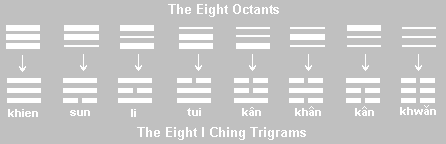# Quantum Alchemy: The Wave Function in Terms of the Four Elements

The Quantum State In Alchemy

by Charles Higgins

The Quantum State is the complementation of three different binary lines. As an example, using the three binary lines, luminosity, humidity, and temperature, this complementation produces a three-dimensional model with the binary lines now labeled p, q and m.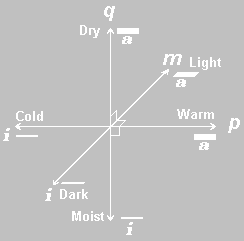Instead of four elements as in the First Complement State, the Quantum State has eight elements. Each one of these eight elements is a complementation of active or inactive elements from the three binary lines. The eight elements of the example are light-dry-warm, light-dry-cold, light-moist-warm, dark-dry-warm, dark-moist-warm, dark-dry-cold, light-moist-cold and dark-moist-cold.

The equation for this 3-dimensional model is derived in a similar manner as the two-dimensional equation. Each element in the Quantum State is a quantum field more simply called an octant. The order of the quantum fields (octants) used below is a binary transformation of the most active field to the most inactive field in accord with the expansion of the 3-dimensional binomial. The most active quantum field is the octant with three active conditions.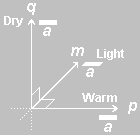In this quantum field the condition is warm, dry and light. The algebraic term is the cross multiplication of an active element from each of the p, q, and m binary lines.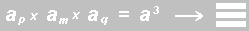In symbol notation, this quantum field has three bold lines positioned one above the other just as in the two-dimensional diagram where the symbols were placed one above the other.

There is also a special order in the placement of the symbols. In the 2-dimensional diagram, the p binary line was placed above the q binary line.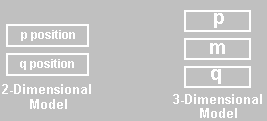In the three-dimensional model the m binary line is placed between the p and q binary lines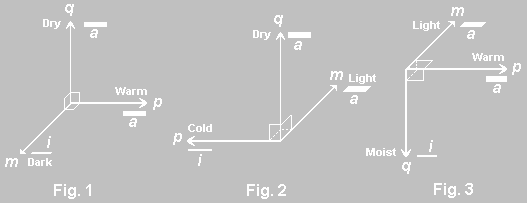The next three quantum fields, shown above, each have two active elements and one inactive element. The first diagram shows a condition of warm, dry and dark. Warm and dry are the active elements of the temperature and humidity binary lines and dark is the inactive element of the luminosity binary line. The algebraic and symbolic term for this quantum field is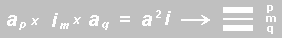The a squared i element is the cross multiplication of the active elements of the p and q binary lines and the inactive element of the m binary line.

The diagram shown in Fig. 2 has a condition of light, dry and cold. The algebraic and symbolic term of this quantum field is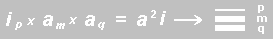This a squared i product is the cross multiplication of the active elements of the m and q binary lines and the inactive element of the p binary line.

The quantum field in Fig.3 is a condition of warm, light and moist, and its algebraic and symbolic term is shown as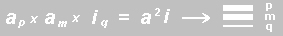The a squared i product of this quantum field is the cross multiplication of the inactive element of the q binary line and the active elements of the m and p binary lines.

The next three quantum fields each have two inactive elements and one active element from the three binary lines.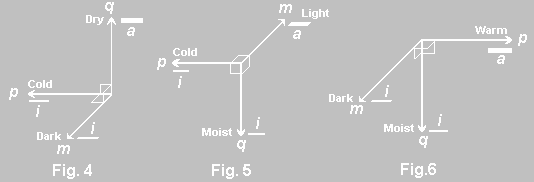The algebraic and symbolic term for each of the three is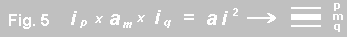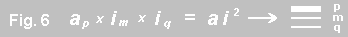The eighth and last quantum field is the product of the cross multiplication of the three inactive elements of the p, q and m binary lines.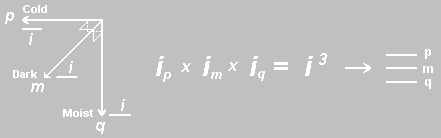The algebraic and symbolic notation for the eight quantum fields is shown by their different combinations of bold and thin lines.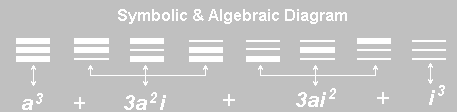The diagram above also shows the algebraic summation of the eight quantum fields. This summation can be shown by the mensional equation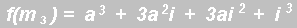and by factoring this summed equation, we arrive at the general equation for the three-dimensional or Quantum State.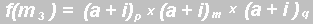or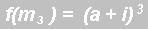The cross multiplication of three additive inverse binary lines is the limit to which we can visually comprehend a diagram represented graphically. Consequently this three-dimensional model is called the quantum state, it is one quantum of complementation. The alchemists referred to this structure as Ether.

The four elements of the First Complement State plus this structure and its complementations the alchemists called the Quintessence of Matter. They believed that all things could be explained by the five elements of the quintessence of matter, Ether, Fire, Earth, Air and Water.

I CHING CORRELATION

From the four emblematic symbols, the eight trigrams are formed. This is true as will be seen in the section on The Alchemist’s Ether, but it is not simply by the addition of another line to the bigrams. First, the hexagram is formed by the addition of three emblematic symbols and then the hexagram is divided into two trigrams for its interpretations. The eight quantum fields of the ether discussed above correspond to the trigrams of the I Ching as shown below.